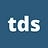A.I. Tools

# Overview of Sorting Algorithms: Quicksort | by Vyacheslav Efimov

## Discover one of the most efficient sorting algorithmsQuicksort is probably the most popular sorting algorithm. In many experiments, it was shown that on average it performs better than other sorting methods like merge sort or heap sort.

Quicksort is based on the idea of recursively splitting an array into two parts: the first which contains all the elements that are less than a pivot element and the second which consists of greater elements. This procedure is called partition. The partition function is recursively called on both divided parts of the array until there is only one element to be partitioned.

Initially, it is necessary to choose a pivot element. This can be any element of the array. In our example, we will choose the last array element. Then we will go through a loop where on each iteration we will compare the current element to the pivot. If the element is less or equal to the pivot, then it will be kept in the left part of the array. Otherwise, the element will be moved to the right one.

First of all, we initialise two pointers: i and j. Index j points to the first element while i points to its precedent value j – 1. With introduced variables i and j, the following invariants are satisfied throughout the loop:

Elements with indices [left, i] are less or equal to the pivot.Elements with indices [i + 1, j) are greater than the pivot.

By the end of the loop, j will be equal to right and index i will divide the array into two parts. Due to the true invariants, our array will be correctly partitioned.

On each iteration, j is incremented by 1, so it always points to the current element. Then the current element array[j] is compared to the pivot. If the element is less or equal to the pivot, then it is swapped with the element at position i + 1. According to the invariant mentioned above, we always know that array[i + 1] > pivot. As a result, the element that is less or equal to the pivot is swapped in the left direction with an element that is greater than the pivot. By doing such a swap our invariant is violated. To make the invariant correct after this, i is incremented by 1.

If array[j] > pivot, then the invariant is still correct and we simply proceed to the next iteration (i does not change its value).

It is easy to notice that after all iterations the array will be correctly partitioned. The described logic is demonstrated in the code snippet below. Notice that the index of the pivot element is returned.

The sorting algorithm proceeds recursively. Firstly, the array is partitioned into two parts, according to the previous section. For each of them, the partition procedure is recursively invoked again until the moment when there is only 1 element to be partitioned.

The function for this algorithm is shown below.

Since the precise asymptotic proof for quicksort includes too many details and is relatively complex, I would not like to dive into all the aspects but provide informal proof.

During each partition call, we linearly pass through all the elements of an array which results in O(K) complexity where K is the array size. Let us assume that after each partition procedure, the pivot element separates an array into two equal halves, so we recursively call the quicksort function for both of them until there is only 1 element left to be sorted. That results in the following function call structure that looks the same for the merge sort.

The only difference is that instead of the merge function, we call the partition function but both of them have the same time complexity O(K). Since the hierarchy structure is also the same, we summarise that the time complexity for quicksort is O(N * logN). The asymptotic proof for the merge sort can be found here.

In the proof above we assumed that the array is always partitioned by two equal halves which is, obviously, not always true. Assume the partition procedure always separates only one element from others. This will result in array sizes of N – 1, N – 2, N – 3, …, 1 on all iterations for which the partition procedure will be invoked. Let us calculate the total time complexity for this scenario by using the summation formula for the arithmetic progression.

T(N) = O(N) + O(N – 1) + O(N – 2) + O(N – 3) + … + O(1) = O(N * (N + 1) / 2) = O(N²)

As a result, we end up with squared time complexity. This is the worst possible case for the quicksort. Despite this, the probability of this scenario is very small.

On average, quicksort works for O(N * logN) time and outperforms other sorting algorithms.

Imagine you develop a system that uses a quicksort algorithm under the hood. It would not be a good idea to always choose a pivot element, according to the same principle (in our example, we were constantly choosing the end element of the array). If somebody finds out the strategy of choosing a pivot element in the system, then this person can expressly input arrays that will result in worst partition scenarios and, as a consequence, in O(N²) time complexity. It might significantly decrease the system’s efficiency. This is why it is always better to choose pivots in different ways for every iteration. One of the most popular ways to do it is to choose a pivot randomly.

We have studied quicksort — a well-known algorithm for sorting. Despite having quadratic complexity in the worst scenario, it still performs usually better in practice, compared to other algorithms like merge sort or heap sort, especially in the case of large arrays.

All images unless otherwise noted are by the author